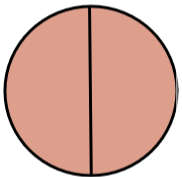# 1.1: Understanding Fractions

$$\newcommand{\vecs}{\overset { \rightharpoonup} {\mathbf{#1}} }$$ $$\newcommand{\vecd}{\overset{-\!-\!\rightharpoonup}{\vphantom{a}\smash {#1}}}$$$$\newcommand{\id}{\mathrm{id}}$$ $$\newcommand{\Span}{\mathrm{span}}$$ $$\newcommand{\kernel}{\mathrm{null}\,}$$ $$\newcommand{\range}{\mathrm{range}\,}$$ $$\newcommand{\RealPart}{\mathrm{Re}}$$ $$\newcommand{\ImaginaryPart}{\mathrm{Im}}$$ $$\newcommand{\Argument}{\mathrm{Arg}}$$ $$\newcommand{\norm}{\| #1 \|}$$ $$\newcommand{\inner}{\langle #1, #2 \rangle}$$ $$\newcommand{\Span}{\mathrm{span}}$$ $$\newcommand{\id}{\mathrm{id}}$$ $$\newcommand{\Span}{\mathrm{span}}$$ $$\newcommand{\kernel}{\mathrm{null}\,}$$ $$\newcommand{\range}{\mathrm{range}\,}$$ $$\newcommand{\RealPart}{\mathrm{Re}}$$ $$\newcommand{\ImaginaryPart}{\mathrm{Im}}$$ $$\newcommand{\Argument}{\mathrm{Arg}}$$ $$\newcommand{\norm}{\| #1 \|}$$ $$\newcommand{\inner}{\langle #1, #2 \rangle}$$ $$\newcommand{\Span}{\mathrm{span}}$$$$\newcommand{\AA}{\unicode[.8,0]{x212B}}$$

Fractions are portions of whole numbers consisting of a numerator (or the top number of the fraction) and the denominator (or the bottom number of the fraction.) They are numbers that represent parts of a whole.Figure $$\PageIndex{1}$$: FractionFigure $$\PageIndex{2}$$: Cake Fractions by Acdx is in the public domainFigure $$\PageIndex{3}$$: Circle with Proportions by COC OER is licensed under CC BY 4.0

Fractions are broken down into three classifications:

• Proper - $$\dfrac{3}{4}$$ - Where the numerator is smaller than the denominator
• Improper - $$\dfrac{5}{2}$$ - Where the numerator is larger than the denominator
• Mixed - $$1\dfrac{6}{7}$$ - Where a whole number precedes the fraction

## Exercise 1.1

Identify the following as a proper fraction, improper fraction, or mixed number. Identify the correct word.

 $$\dfrac{1}{2}$$ Proper Improper Mixed $$\dfrac{10}{12}$$ Proper Improper Mixed 3. $$10 \dfrac{1}{2}$$ Proper Improper Mixed $$\dfrac{11}{3}$$ Proper Improper Mixed $$\dfrac{101}{13}$$ Proper Improper Mixed $$130 \dfrac{10}{13}$$ Proper Improper Mixed $$\dfrac{1,000}{1,111}$$ Proper Improper Mixed $$\dfrac{12}{7}$$ Proper Improper Mixed $$\dfrac{1}{7}$$ Proper Improper Mixed $$13 \dfrac{1}{7}$$ Proper Improper Mixed

1.1: Understanding Fractions is shared under a CC BY license and was authored, remixed, and/or curated by LibreTexts.